Series Parallel Operation of SCR

# Series Parallel Operation of SCR - Notes | Study Electrical Engineering SSC JE (Technical) - Electrical Engineering (EE)

 1 Crore+ students have signed up on EduRev. Have you?

## Series  Parallel Operation of SCR

Series / Parallel Operation of SCR

• When single SCR is not sufficient to provide required voltage or current rating, then more SCR's, are connected in series (to meet higher voltage demand) or in parallel (to meet higher current demand).
• In series or parallel operation, SCRs are not utilized fully (as characteristics of SCR are not matched exactly). To measure the degree of utilization of thyristors string efficiency (x) is used and defined as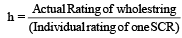* (Number of SCRs in string)

When η = 1 each SCR in string has 100% identical characteristic or utilization of thyristor will be maximum. But it is not possible practically.

• Derating factor : A measure of the reliability of string is given by a factor called derating factor. DRF = 1 – η

Series Operation

• Series connected SCRs (1) and (2) carry same current IO but due to unmatched static characteristic as shown in figure voltages are different say V1 and V2. So, h = V1 + V2/2V1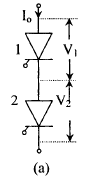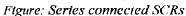•  This shows that even though SCRs have identical ratings, voltage shared by each is not the same and string efficiency is therefore less than one.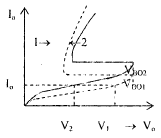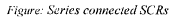• A uniform voltage distribution in steady state can be achieved by connecting suitable resistance across each SCR. A more practical way of obtaining a reasonably uniform voltage distribution during steady state of series connected SCRs to connect the same value of shunt resistance R across each SCR as shown in figure below.

• For the sake of simplicity, let us consider out of the string of n SCRs, only 1st is different. Let leakage current for SCR be Ibmn and for remaining (n-1) SCRs be Ibmx such as Ibmx > Ibmn
Let string current be I
Hence I1 = I – Ibmn and I2 I – Ibms
Voltage across SCRI is Vbm = I1R

Voltage across each of remaining (n – 1) SCRs = (n – 1) I2R

For a string voltage Vs, the voltage equation for the series circuit will be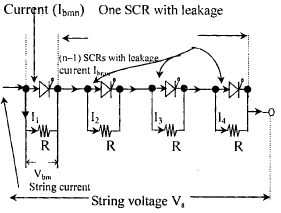Vs = I1 R + (n –1)I2 R

= Vbm + (n – 1) R [I1 – (Ibmx – Ibmn)]

let (Ibmx – Ibmn) = ΔIb

RI1 = Vbm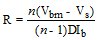• Not only the static characteristics need to be identical but also the transient or dynamic characteristic.
Identical dynamic characteristic means equalization of potential is needed during turning on and tuning off periods. These periods may not be exactly equal for all the thyristors connected in series.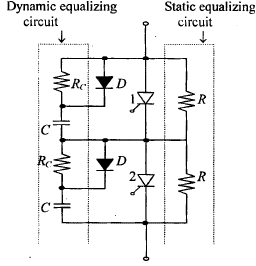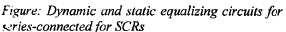• To equalize these transient voltages, a dynamic equalizing circuit consisting of a capacitor is connected across each SCR is used.
• The Rc connected in parallel with Diode D in dynamic equalizing circuit are provided to limit discharging current of capacitor through SCR.

Parallel Operation

When current required by the load is more than the rated current of a single thyristor, SCRs are connected in parallel in string. For equal sharing of currents V-I characteristics of each SCRs during forward conduction must be identical as far as possible.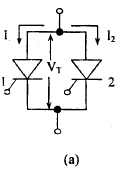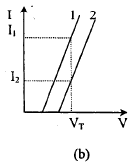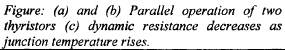For parallel-connected SCRs, voltage VT across them must be equal. Figure (b) show that for a same voltage drop VT, SCR1 shares a rated current I1 whereas SCR2 carries current I2 much less than rated current I1. The total current carried by the unit is I1 + I2 and not the rated current 2I1 as required. Therefore string efficiency is given by: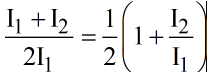• When SCRs are connected in parallel, all units must operate at the same temperature as far as possible. This is done by having a common heat sink.
• Unequal current distribution in a parallel unit is also caused by the inductive effect of current carrying conductors. This unequal current distribution can be avoided by mounting the SCRs symmetrically on the heat sink.

The document Series Parallel Operation of SCR - Notes | Study Electrical Engineering SSC JE (Technical) - Electrical Engineering (EE) is a part of the Electrical Engineering (EE) Course Electrical Engineering SSC JE (Technical).
All you need of Electrical Engineering (EE) at this link: Electrical Engineering (EE)

## Electrical Engineering SSC JE (Technical)

2 videos|75 docs|37 tests

## Electrical Engineering SSC JE (Technical)

2 videos|75 docs|37 tests

Track your progress, build streaks, highlight & save important lessons and more!

,

,

,

,

,

,

,

,

,

,

,

,

,

,

,

,

,

,

,

,

,

;